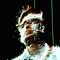Building a Vector Space Search Engine in Python

A vector space search involves converting documents into vectors. Each dimension within the vectors represents a term. If a document contains that term then the value within the vector is greater than zero.

Here is an implementation of Vector space searching using python (2.4+).

1 Stemming & Stop words

Fetch all terms within documents and clean – use a stemmer to reduce. A stemmer takes words and tries to reduce them to there base or root. Words which have a common stem often have similar meanings. Example: CONNECTED CONNECTING CONNECTION CONNECTIONS

all map to CONNECT

We also remove any stopwords from the documents. [a,am,an,also,any,and] are all examples of stopwords in English. Stop words have little value in search so we strip them. The stoplist used was taken from: ftp://ftp.cs.cornell.edu/pub/smart/english.stop

self.stemmer = PorterStemmer()

2 Map Keywords to Vector Dimensions

Map the vector dimensions to keywords using a dictionary: keyword=>position

3 Map Document Strings to Vectors.

We use the simple Term Count Model. A more accurate model would be to use tf-idf (termFrequency-inverseDocumentFrequnecy).

4 Find Related Documents

We now have the ability to find related documents. We can test if two documents are in the concept space by looking at the the cosine of the angle between the document vectors. We use the cosine of the angle as a metric for comparison. If the cosine is 1 then the angle is 0° and hence the vectors are parallel (and the document terms are related). If the cosine is 0 then the angle is 90° and the vectors are perpendicular (and the document terms are not related).

We calculate the cosine between the document vectors in python using scipy.

5 Search the Vector Space!

In order to perform a search across keywords we need to map the keywords to the vector space. We create a temporary document which represents the search terms and then we compare it against the document vectors using the same cosine measurement mentioned for relatedness.

Further Extensions

1. Use tf-idf rather than the Term count model for term weightings

2. Instead of linear processing of all document vectors when searching for related content use: Lanczos methods OR a neural network-like approach.

Third Party tools

The stemmer used comes from: http://tartarus.org/~martin/PorterStemmer/python.txt

And the library for performing cosine calculations comes from NumPy: http://www.scipy.org/

Source

https://github.com/josephwilk/semanticpy.git